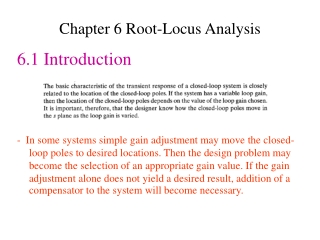DownloadDownload PresentationChapter 6 Root-Locus Analysis

# Chapter 6 Root-Locus Analysis

Download Presentation## Chapter 6 Root-Locus Analysis

- - - - - - - - - - - - - - - - - - - - - - - - - - - E N D - - - - - - - - - - - - - - - - - - - - - - - - - - -
##### Presentation Transcript

1. Chapter 6 Root-Locus Analysis 6.1 Introduction - In some systems simple gain adjustment may move the closed-loop poles to desired locations. Then the design problem may become the selection of an appropriate gain value. If the gain adjustment alone does not yield a desired result, addition of a compensator to the system will become necessary.

2. Unless otherwise stated, we shall assume that the gain of the open-loop transfer function is the parameter to be varied through all values, from zero to infinity. - By using the root-locus method the designer can predict the effects on the location of the closed-loop poles of varying the gain value or adding open-loop poles and/or open-loop zeros.

3. - The values of s that fulfill both the angle and magnitude conditions are the roots of the characteristic equation, or the closed-loop poles. • A locus of the points in the complex plane satisfying the angle condition alone is the root locus. - The roots of the characteristic equation (the closed-loop poles) corresponding to a given value of the gain can be determined from the magnitude condition.

4. - Begin sketching the root loci of a system by the root-locus method.For example, if G(s)H (s) is given by

5. Figure 6-2 (a) and (b) Diagrams showing angle measurements from open-loop poles and open-loop zero to test point s.

6. Figure 6-3 Control system.

7. - The number of individual root loci for this system is three, which is the same as the number of open-loop poles.

8. Figure 6-4 Three asymptotes.

9. Figure 6-5 Construction of root locus.

10. Figure 6-6 Root-locus plot.

11. Figure 6-7 Control system.

12. Figure 6-9 Determination of the angle of departure.

13. - If a root locus lies between two adjacent open-loop poles on the real axis, then there exists at least one breakaway point between the two poles. - If the root locus lies between two adjacent zeros (one zero may be located at -) on the real axis, then there always exists at least one break-in point between the two zeros. -If the root locus lies between an open-loop pole and a zero (finite or infinite) on the real axis, then there may exist no breakaway or break-in points or there may exist both breakaway and break-in points.

14. - The angle of departure (or angle of arrival) of the root locus from a complex pole (or at a complex zero) can be found by subtracting from 180 the sum of all the angles of vectors from all other poles and zeros to the complex pole (or complex zero) in question, with appropriate signs included.

15. Figure 6-12 Construction of the root locus. [Angle of departure =180°- (ϴ1+ϴ2 )+Φ.]

16. Figure 6-15 Control system.

17. Figure 6-17 Root-locus plot.

18. Figure 6-18 Root-locus plot.

19. Figure 6-19 Closed-loop control system.

20. Figure 6-20 Root-locus plot of system defined in state space, where A, B, C, and D are as given by Equation (6–15).